# Goodwin Model (1967)

A few words about the model. Consider that the production function has complementary factors according to the following equation:we know that such an assumption is useful to engender fluctuations. The effective labor at the period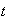is denoted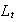but the working population isso that the labor rate is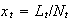. The wage depends on the labor rate according to an assumption of the Philips curve type. The profits are entirely reinvested, they are equal to the savings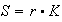and the part of profits in the global income is the complement of the part of wagesso that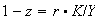. The labor depends on the capital according to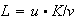. Then we have the system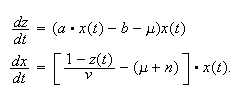There is stationary state if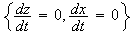, i.e.,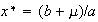and*

 ref. to Alain Goergen. "Dynamique Economique. Solutions de problèmes avec Maple et Matlab". Economica. 2006.

 ref. to Gilbert Abraham-Frois.